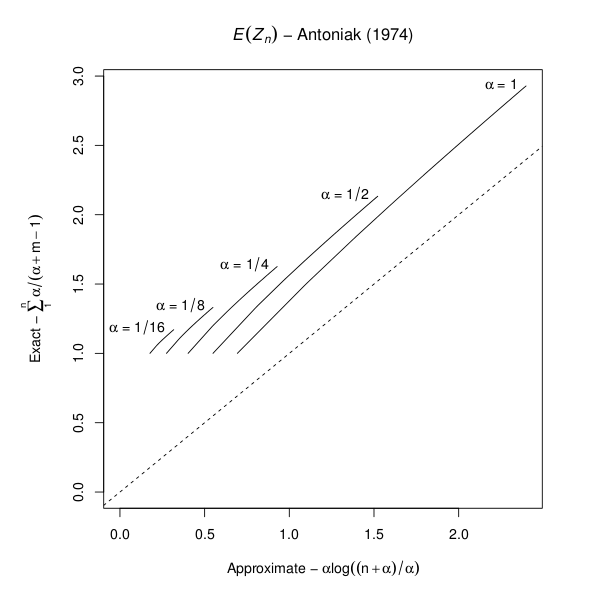# A Note on Antoniak's Approximation for Dirichlet Processes

Antoniak's 1974 article titled Mixtures of Dirichlet Processes with Applications to Bayesian Nonparametric Problems (Annals of Statistics 2(6):1152-1174) is a fundamental work for most modern developments in this area. The article gives two expressions for the expected number of distinct values in a sample of size , drawn from a Dirichlet process-distributed probability distribution with precision parameter . The first expression is exact, and the second is approximate. However, I recently discovered (the 'hard way') that the approximation is poor for . The following R graphic illustrates the disparity for several values of  and .### R Code

approx <- function(alpha, n) alpha * log((1:n + alpha)/alpha)
exact <- function(alpha, n) cumsum(alpha/(alpha + 1:n - 1))
approx_exact_plot <- function(alp, n, ...) {
amax <- max(1/alp)
appmax <- approx(amax, n)
examax <- exact(amax, n)
par(mar = c(5, 6, 4, 3) + 0.1)
plot(appmax, examax, type = "l", xlim = c(0, max(appmax)),
ylim = c(0, max(examax)),
main = expression(paste(italic(E)(italic(Z)[italic(n)]), " - Antoniak (1974)")),
xlab = expression(paste("Approximate - ", alpha, "log", ((n + alpha)/alpha))),
ylab = expression(paste("Exact - ", sum(alpha/(alpha + m - 1), 1, n))), ...)
text(max(appmax), max(examax), substitute(paste(alpha, " = ",
amax)), pos = 2)
abline(a = 0, b = 1, lty = 2)
if (length(alp) == 1)
return()
for (a in alp[-1]) {
app <- approx(1/a, n)
exa <- exact(1/a, n)
points(app, exa, type = "l")
text(max(app), max(exa), substitute(paste(alpha, " = ",
1/a)), pos = 2)
}
}
approx_exact_plot(2^(0:4), 10)